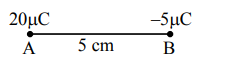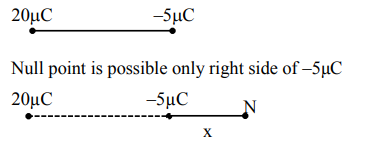# Two particles A and B having chargesQuestion:

Two particles A and B having charges $20 \mu \mathrm{C}$ and $-5 \mu \mathrm{C}$ respectively are held fixed with a separation of $5 \mathrm{~cm}$. At what position a third charged particle should be placed so that it does not experience a net electric force?1. At $5 \mathrm{~cm}$ from $20 \mu \mathrm{C}$ on the left side of system

2. At $5 \mathrm{~cm}$ from $-5 \mu \mathrm{C}$ on the right side

3. At $1.25 \mathrm{~cm}$ from $-5 \mu \mathrm{C}$ between two charges

4. At midpoint between two charges

Correct Option: , 2

Solution:$\mathrm{E}_{\mathrm{N}}=+\frac{\mathrm{k}(-5 \mu \mathrm{C})}{\mathrm{x}^{2}}+\frac{\mathrm{k}(20 \mu \mathrm{C})}{(5+\mathrm{x})^{2}}=0$

$x=5 \mathrm{~cm}$

$\therefore$ option (2) is correct